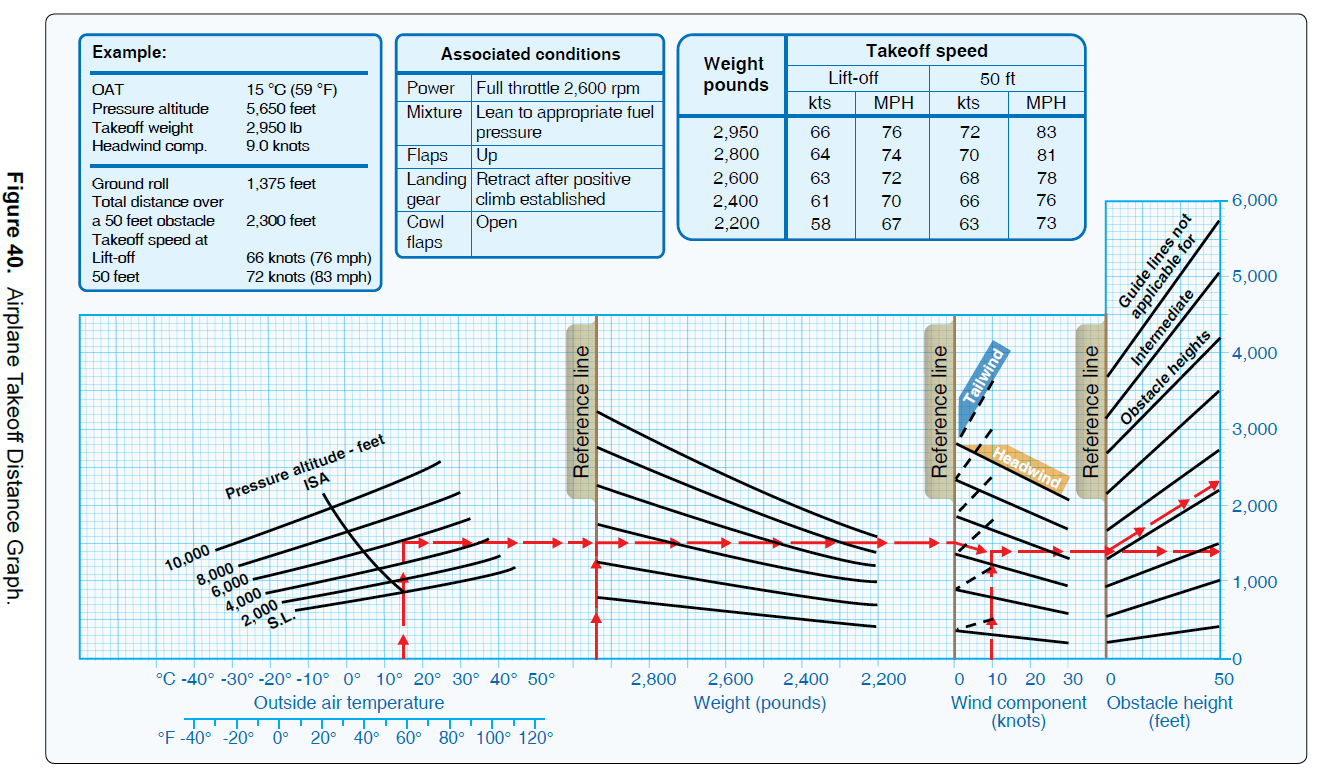# Quiz: Performance and Limitations 1

0

Performance and Limitations 1

PA.I.F.K1 Elements related to performance and limitations by explaining the use of charts, tables, and data to determine performance.

1 / 28

1.

Determine the total distance required to land over a 50-foot obstacle. (Refer to Figure 38: Airplane Landing Distance Table)
Pressure altitude = 7,500 ft
Temperature = 32°F
Runway = Hard surface

2 / 28

2.

Determine the total distance required to land over a 50-foot obstacle. (Refer to Figure 38: Airplane Landing Distance Table)
Pressure altitude = 5,000 ft
Temperature = 41°F
Runway = Hard surface

3 / 28

3.

Determine the approximate landing ground roll distance. (Refer to Figure 38: Airplane Landing Distance Table)
Pressure altitude = 1,250 ft
Temperature = Std

4 / 28

4.

Determine the approximate landing ground roll distance. (Refer to Figure 38: Airplane Landing Distance Table)
Pressure altitude = 5,000 ft
Temperature = 101°F

5 / 28

5.

Determine the total distance required to land over a 50-ft. obstacle. (Refer to Figure 38: Airplane Landing Distance Table)
Pressure altitude = 3,750 ft
Temperature = Std

6 / 28

6.

Determine the approximate landing ground roll distance. (Refer to Figure 38: Airplane Landing Distance Table)
Pressure altitude = Sea level
Temperature = Std

7 / 28

7.

Determine the total distance required to land. (Refer to Figure 37: Airplane Landing Distance Graph)
OAT = 32°F
Pressure altitude = 8,000 ft
Weight = 2,600 lb
Obstacle = 50 ft

8 / 28

8.

Determine the total distance required to land. (Refer to Figure 37: Airplane Landing Distance Graph)
OAT = 90°F
Pressure altitude = 3,000 ft
Weight = 2,900 lb
Obstacle = 50 ft

9 / 28

9.

Determine the approximate total distance required to land over a 50-ft. obstacle. (Refer to Figure 37: Airplane Landing Distance Graph)
OAT = 90°F
Pressure altitude = 4,000 ft
Weight = 2,800 lb

10 / 28

10.

Determine the total distance required to land. (Refer to Figure 37: Airplane Landing Distance Graph)
OAT = Std
Pressure altitude = 10,000 ft
Weight = 2,400 lb
Wind component = Calm
Obstacle = 50 ft

11 / 28

11. With a reported wind of south at 20 knots, which runway is appropriate for an airplane with a 13-knot maximum crosswind component? (Refer to Figure 36: Crosswind Component Graph)

12 / 28

12. What is the maximum wind velocity for a 30° crosswind if the maximum crosswind component for the airplane is 12 knots? (Refer to Figure 36: Crosswind Component Graph)

13 / 28

13. With a reported wind of north at 20 knots, which runway is acceptable for use for an airplane with a 13-knot maximum crosswind component? (Refer to Figure 36: Crosswind Component Graph)

14 / 28

14. Determine the maximum wind velocity for a 45° crosswind if the maximum crosswind component for the airplane is 25 knots. (Refer to Figure 36: Crosswind Component Graph)

15 / 28

15. What is the headwind component for a landing on Runway 18 if the tower reports the wind as 220° at 30 knots? (Refer to Figure 36: Crosswind Component Graph)

16 / 28

16. What is the crosswind component for a landing on Runway 18 if the tower reports the wind as 220° at 30 knots? (Refer to Figure 36: Crosswind Component Graph)

17 / 28

17. Approximately what true airspeed should a pilot expect with full throttle at 10,500 feet with a temperature of 36°F above standard? (Refer to Figure 35: Airplane Power Setting Table)

18 / 28

18. Approximately what true airspeed should a pilot expect with 65 percent maximum continuous power at 9,500 feet with a temperature of 36°F below standard? (Refer to Figure 35: Airplane Power Setting Table)

19 / 28

19. Determine the approximate manifold pressure setting with 2,450 RPM to achieve 65 percent maximum continuous power at 6,500 feet with a temperature of 36°F higher than standard. (Refer to Figure 35: Airplane Power Setting Table)

20 / 28

20.

What is the expected fuel consumption for a 500-nautical mile flight under the following conditions? (Refer to Figure 35: Airplane Power Setting Table)
Pressure altitude = 4,000 ft
Temperature = +29°C
Manifold pressure = 21.3" Hg
Wind = Calm

21 / 28

21.

What is the expected fuel consumption for a 1,000-nautical mile flight under the following conditions? (Refer to Figure 35: Airplane Power Setting Table)
Pressure altitude = 8,000 ft
Temperature = 22°C
Manifold pressure = 20.8" Hg
Wind = Calm

22 / 28

22. What fuel flow should a pilot expect at 11,000 feet on a standard day with 65 percent maximum continuous power? (Refer to Figure 35: Airplane Power Setting Table)

23 / 28

23.

Determine the approximate ground roll distance required for takeoff. (Refer to Figure 40: Airplane Takeoff Distance Graph)
OAT = 32°C
Pressure altitude = 2,000 ft
Takeoff weight = 2,500 lb

24 / 28

24.

Determine the total distance required for takeoff to clear a 50-foot obstacle. (Refer to Figure 40: Airplane Takeoff Distance Graph)
OAT = Std
Pressure altitude = 4,000 ft
Takeoff weight = 2,800 lb

25 / 28

25.

Determine the total distance required for takeoff to clear a 50-foot obstacle. (Refer to Figure 40: Airplane Takeoff Distance Graph)
OAT = Std
Pressure altitude = Sea level
Takeoff weight = 2,700 lb26 / 28

26.

Determine the approximate ground roll distance required for takeoff. (Refer to Figure 40: Airplane Takeoff Distance Graph)
OAT = 38°C
Pressure altitude = 2,000 ft
Takeoff weight = 2,750 lb27 / 28

27. Where may an aircraft’s operating limitations be found if the aircraft has an Experimental or Special light-sport airworthiness certificate?

28 / 28

28. Where may an aircraft’s operating limitations be found?